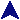Eight-BIt AdderRuby Example Code#
# This is a one-bit adder.
#

require "csim"
require "cgrp"
require "oba"

NumberOut.shush

# Blueprint for a the one-bit adder
bp = OBA.new

# Two input senders, and the output device.
na = SwitchBank.new
nb = SwitchBank.new
disp = NumberOut.new("  Sum")

# We're going to build an 8-bit adder
prev = nil
8.times do
# Create the one-bit adder and join the data inputs and outputs.

# Chain the carry, if this isn't he first one.
if prev then
end

end

# Overflow light.
prev.join(LED.new("  Oflow"))

NumberOut.shush(false)

30.times do
a = rand(256)
b = rand(256)
print a, " + ", b, ":\n"
Gate.activate
na.value = a
nb.value = b
Gate.deactivate
end